Next: REFERENCES Up: Claerbout: Data gap filling Previous: RESEARCH GOALS

# 3-D EXTENSION PROPOSAL

Consider three seismograms on the earth surface that are not on a line. Let the seismograms be denoted as A, B, and C. Let seismogram A be located at ra=(xa,ya) with a likewise location for B and C. Take a monoplane model,.Define three times which are linear functions of px and py.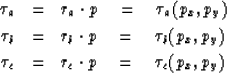(1) (2) (3)
Let Z be the familiar delay operator of Z-transform theory. Now, do a global scan over the two parameters (px, py) where for each value of (px, py) you solve the following minimization of the quadratic in three scalar variables.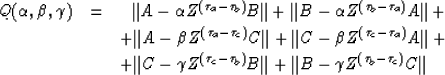(4)
where summation over a small time gate is denoted by.Absorbing the time shifts into the definitions of A, B, and C and minimizing Q by variation ofleads to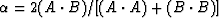with similar expressions for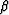and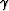.

The value of (px,py) is found by the global search of Q(px,py). A two-dimensional search on (px,py) space is not as costly as it sounds. We can first run burone()for each of the three rows in equation (4) for all possible one-dimensional stepouts and tabulate the outputs. Then the two-dimensional global search requires only a few additions from the tables for each point in (px,py) space.

If we were to follow the philosophy of my 2-D code, the value of the interpolated trace is far less important than the fact that we have used the (px,py) from above. In other words, we can throw away.The value of the unknown trace could be(5)
where the wi depend only on geometry and the scale, if any, is the overall coherence, say.Alternately, it is not difficult to make more elaborate models.There are many ways to bake the cake. I believe, however, the most important feature of any formulation is that it should maintain a very minimal number of adjustable parameters because we want to go to very small time gates. If the original data were on a regular rectangular mesh, instead of an arbitrary three locations, then we can reduce the three free parameters above to two. First, permit me to change variables so all the values of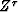are unity. Then usefor north-south correlation andfor east-west correlation.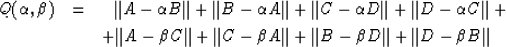(6)Next: REFERENCES Up: Claerbout: Data gap filling Previous: RESEARCH GOALS
Stanford Exploration Project
11/18/1997